# (Solutions) Chemical Kinetics Class 12 Test – 1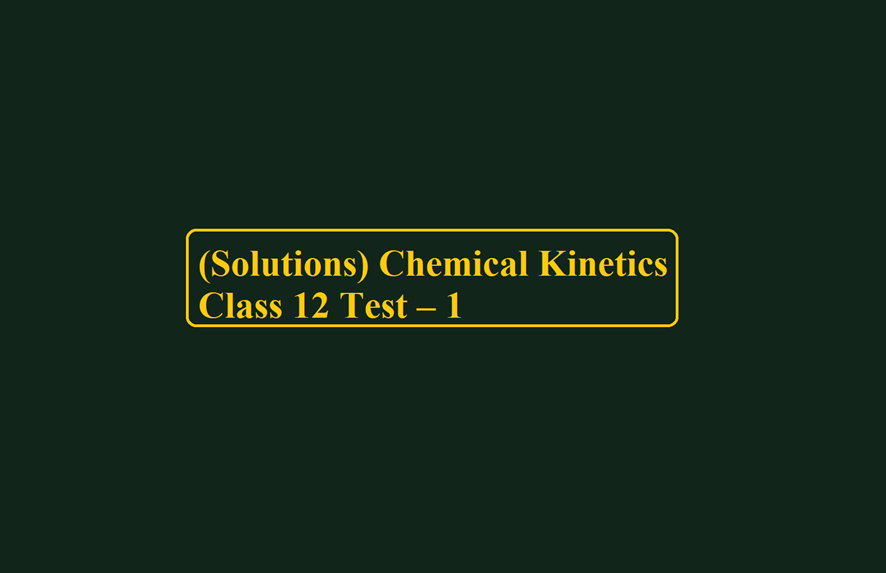(Solutions) Chemical Kinetics Class 12 Test – 1

Chemical Kinetics Class 12

Ans 1. (B) Rate is inversely proportional to the surface coverage

Ans 2. (A). V0 ∝ a
Vt ∝ a + x
Vt – V0 ∝ (a + x – a)
2V0 – Vt ∝ a – x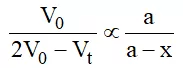Ans 3.  (B). The rate law for the dimerization of NO2 is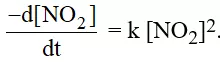The temperature will change the value of the specific rate constant.

Ans 4. (C). According to the Arrhenius equation, a straight line is to be obtained by plotting the logarithm of the rate constant of a chemical reaction (log k) against 1/T.

Ans 5. (C). The rate of reaction can be increased by increasing the conc. of both Nu and R – X

Ans 6. (A).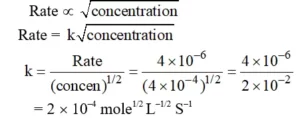Ans 7. (B). For the reaction 2NO + O2 → 2NO2, when the volume of the reaction vessel is doubled rate reduces to one-eights of its initial rate.

Ans 8. (B). The molecularity of the reaction is 3/2

Ans 9. (D).   For the reaction, H2(g) + Br2(g) → 2HBr(g),
The reaction rate = K [H2] [Br2]1/2
The Molecularity of the reaction is 2.

Ans 10. (C). This is a gaseous phase reaction.  One mole of gaseous ether decomposes to give three moles of gaseous products.
pi = initial pressure of ether
x = decrease in pressure of ether after time t
3x = pressure of three gaseous products after time t
∴ Total pressure at the time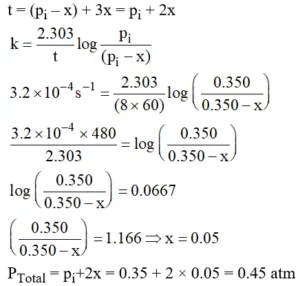Chemical Kinetics Class 12

Ans 11. (C). According to k = Ae–Ea/RT (Arrhenius equation), the activation energy of a reaction in the presence of enzymes is different from Ea obtained in the laboratory.

Ans 12. (B). The chemical reaction, 2O3 → 3O2 proceeds as follows:
O3 → O2 + O        …… (fast)
O + O3 → 2O2  …… (slow)
The rate law expression is
r = K[O3]2 [O2]–1

Ans 13. (B). The time required to decompose half of the substance for the nth-order reaction is inversely proportional to an–1.

Ans 14. (D). The overall reaction order is 3 + 0 = 3.

Ans 15. (B). For the elementary step,
(CH3)3.CBr(aq) → (CH3)3 C+(aq) + Br¯ (aq)
The molecularity is 1.

Ans 16. (B). For a reaction for which the activation energies of the forward and reverse directions are equal in value ΔH = 0.

Ans 17. (D). If the concentrations are doubled, then the reaction rate will increase by a factor of 22 × 21/2 = 5.7.

Ans 18. (A). The Arrhenius eq. is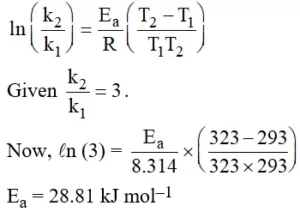Ans 19. (D). The initial rate is used so that the observed rate will not depend on product concentration.

Ans 20. (B). The unit for each concentration factor on the right side of the rate expression is M, so,
Rate = M/s = k (M)(M)3/2.
k must have units of 1/(min. M3/2) to produce M/s as the overall unit.

Ans 21. (C). The filled shells will repel one another.

Ans 22. (C)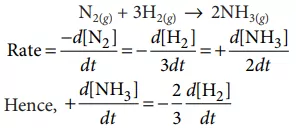Ans 23. (D). Collision frequency ∝ no. of reacting molecules or atoms Higher the concentration of reactant molecules, the higher is the probability of collision and so the collision frequency

Ans 24. (A). If for any reaction, the rate constant is equal to the rate of the reaction at all concentration then the order is zero.

Ans 25. (B).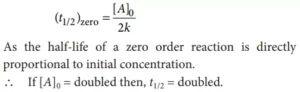Ans 26. (D). Since the enzymes are regarded as biological catalysts, therefore their action increases the rate of biological reaction.

Ans 27. By seeing the curve, activation energy for backward reaction = 100 kJ.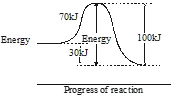Ans 28. The rate for the reaction: A + B + C → Product
rate = k[A]1/2 [B]1/4 [C]0
The order of the reaction is 3/4.

Ans 29. If the rate expression for the reaction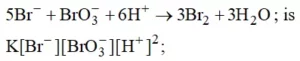the order of the reaction is 4.

Ans 30. The elementary step of the reaction,
2Na + Cl2 → 2NaCl is found to follow III order kinetics, its molecularity is 3.

Chemical Kinetics Class 12